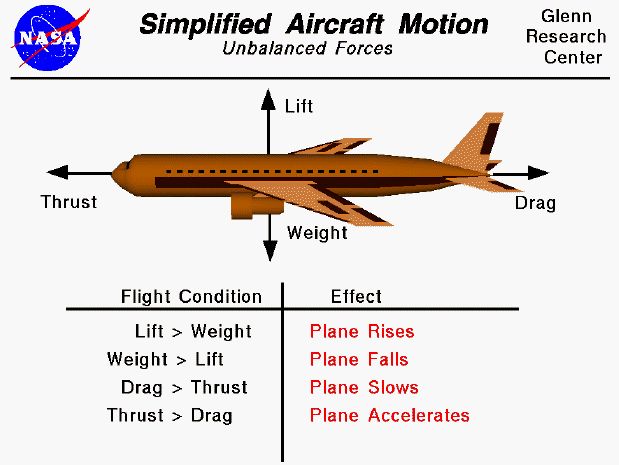This slide shows some rules for the simplified motion of an aircraft. By simplified motion we mean that some of the four forces acting on the aircraft are balanced by other forces and that we are looking at only one force and one direction at a time. In reality, this simplified motion doesn't occur because all of the forces are interrelated to the aircraft's speed, altitude, orientation, etc. But looking at the forces ideally and individually does give us some insight and is much easier to understand.

In an ideal situation, an airplane could sustain a constant speed and level flight in which the weight would be balanced by the lift, and the drag would be balanced by the thrust. The closest example of this condition is a cruising airliner. (While the weight decreases due to fuel burned, the change is very small relative to the total aircraft weight.) In this situation, the aircraft will maintain a constant cruise velocity as described by Newton's first law of motion.

If the forces become unbalanced, the aircraft will move in the direction of the greater force. We can compute the acceleration which the aircraft will experience from Newton's second law of motion (F = m a). We must first determine the mass of the aircraft from it's weight, and the force (F) would be the difference between the opposing forces (lift minus weight or thrust minus drag). With this information, we can solve for the resulting motion of the aircraft.

In summary, if the weight is decreased while the lift is held constant, the airplane will rise (Lift > Weight). Or, if the lift is decreased while the weight is constant, the plane will fall (Weight > Lift). Similarly, increasing the thrust while the drag is constant will cause the plane to accelerate (Thrust > Drag). And increasing the drag at a constant thrust will cause the plane to slow down (Drag > Thrust).

Guided Tours